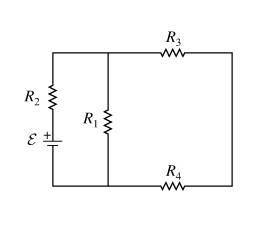# Question concerning Kirchoff's Laws

• Linus Pauling
In summary, according to the laws of series and parallel connections, the relationships between I1, I3, and I4 are: I1 = I3, I3 = I4, and I2 = I3 + I4.

#### Linus Pauling

1. Which of the following relationships must be true according to the laws of series and parallel connections?The options are:
I1 = I3
I3 = I4
I2 = I3 + I4
I1 = I3 + I2
V1 = V2
V3 = V4
V1 = V3 + V4
V2 = V3 + V4
V1 = V2 + V3 + V4

2. Loop and junction laws

3. By the junction law, I said that I1 = I3, I3 = I4, and I2 = I3 + I4. That's wrong, and I suspect that it's wrong because one or more of the options involving the potential drops is correct. But I don't understand why any of those would be true if the potential drops across each resistor in the direction of current flow.

I assume I1 is the current through R1, etc. The truth of some of the answers depends on the values of the resistors.

What does Kirchoff's current law say? Explain how you applied it to get the answers you did.

3 and 4 are in series, so they must have the same current. I mis-typed above: I said I2 = I1 + I3, because current flowing from I2 reaches the junction then goes to either 1 or 3... also, I changed my mind, I1 does not equal I3, I1 is greater because on the "I3" branch there's two resistors.

Linus Pauling said:
3 and 4 are in series, so they must have the same current. I mis-typed above: I said I2 = I1 + I3, because current flowing from I2 reaches the junction then goes to either 1 or 3...
Yes.
Also, I changed my mind, I1 does not equal I3; I1 is greater because on the "I3" branch there are two resistors.
This is one of those cases where the specific resistor values matter. You can't just count the number of resistors. If R1 > R3+R4, then I1 < I3, right?

Right, so I3 = I4 and I2 = I1 + I3... but what else am I missing? I assume one or more of the options regarding potential is correct, but as I stated I don't understand why.

Apply Kirchoff's voltage law to the three loops in the circuit.

Kirchoff's current law tells you that elements in series have the same current; Kirchoff's voltage law tells you that elements in parallel have the same voltage across them.

Note that the problem isn't well stated as both the currents and voltages should have a direction associated with them. I assume they're just working with magnitudes in the equations.